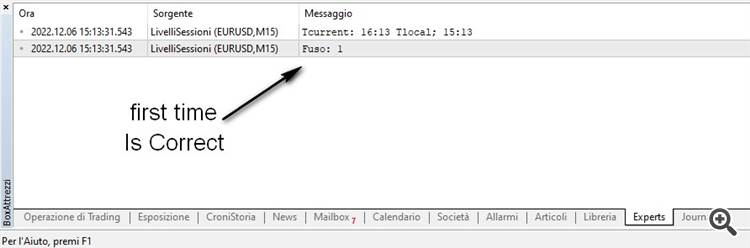# Problem whit reboot MT5

I have a problem restarting MT5 if I load the indicator on the chart the first time everything is ok but if I close and restart MT5 the time is not initialized correctly

```//+------------------------------------------------------------------+
//| Custom indicator initialization function                     |
//+------------------------------------------------------------------+
int OnInit()
{

tt2= (int)TimeLocal();
tt3= tt1-tt2;

if(tt3==3600 ) fuso=1;

Print("Tcurrent: ",TimeToString(tt1,TIME_MINUTES)," Tlocal; ",TimeToString(tt2,TIME_MINUTES));
Print("Fuso: ",IntegerToString(fuso));

return(INIT_SUCCEEDED);
}``````int OnInit(){
don't try to use any price (or indicator) or server related functions in OnInit (or on load or in OnTimer before you've received a tick), as there may be no connection/chart yet:
1. Terminal starts.
2. Indicators/EAs are loaded. Static and globally declared variables are initialized. (Do not depend on a specific order.)
3. OnInit is called.
4. For indicators OnCalculate is called with any existing history.
5. Human may have to enter password, connection to server begins.
6. New history is received, OnCalculate called again.
7. A new tick is received, OnCalculate/OnTick is called. Now TickValue, TimeCurrent, account information and prices are valid.

thanks William Roeder but I tried to enter OnCalculate and only once for each bar and on the first call there is always the same error

```//+------------------------------------------------------------------+
//| Custom indicator iteration function                           |
//+------------------------------------------------------------------+
int OnCalculate(const int rates_total,
const int prev_calculated,
const datetime &time[],
const double &open[],
const double &high[],
const double &low[],
const double &close[],
const long &tick_volume[],
const long &volume[],
{

// NewBar
if(prev_calculated != rates_total)
{

int tt2= (int)TimeLocal();
int tt3= tt1-tt2;

Print("Tcurrent: ",TimeToString(tt1,TIME_MINUTES)," Tlocal; ",TimeToString(tt2,TIME_MINUTES));

if(tt3==3600 ) fuso=1;